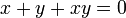# Algebra group

(diff) ← Older revision | Latest revision (diff) | Newer revision → (diff)
This article defines a group property: a property that can be evaluated to true/false for any given group, invariant under isomorphism
View a complete list of group properties
VIEW RELATED: Group property implications | Group property non-implications |Group metaproperty satisfactions | Group metaproperty dissatisfactions | Group property satisfactions | Group property dissatisfactions
WARNING: POTENTIAL TERMINOLOGICAL CONFUSION: Please don't confuse this with algebraic group or group algebra

## Definition

### General definition

Let$N$ be an associative algebra over a field$F$ with the property that for any$x \in N$, there exists$y \in N$ such that$x + y + xy = 0$. (in other words,$N$ is a radical ring).

Then, the algebra group corresponding to$N$ is the adjoint group of$N$, i.e., the group whose elements are formally:$\{ 1 + x | x \in N\}$

where the multiplication is defined as:$(1 + x)(1 + y) = 1 + (x + y + xy)$

There are two interpretations of this:

1. We can think of the algebra group as living inside the unitization$N + F$ as the coset of$N$ for$1 \in F$.
2. Alternatively, since there is a bijection between$N$ and its algebra group, we can think of the algebra group as simply the set$N$ with group operation$x * y := x + y + xy$. This is based on the idea of the multiplicative formal group law. However, unlike other formal group laws, the multiplicative formal group law is valid even in non-commutative settings, and that is necessary for this definition.

### Nilpotent case

If$N$ is an associative algebra over a field$F$ with the property that every element of$N$ is nilpotent, then it is true that for any$x \in N$, there exists$y \in N$ such that$x + y + xy = 0$. Thus, we can always define the algebra group corresponding to any such algebra$N$.

### Finite field case

If$F$ is a finite field and$N$ is a finite-dimensional associative algebra over$F$, the following are equivalent:

1. For any$x \in N$, there exists$y \in N$ such that$x + y + xy = 0$.
2. Every element of$N$ is nilpotent.
3.$N$ is a nilpotent algebra, i.e., there is some fixed length such that all products of that length of elements in$N$ equal zero.

Thus, for a finite-dimensional associative algebra over a finite field$F$, we talk of its algebra group only if it satisfies these equivalent conditions.

### In the context of an abstract group

A group of prime power order which can be realized as an algebra group any finite field is termed an algebra group. Note that an algebra group over$\mathbb{F}_q$, where$q$ is a power of a prime$p$, must also be an algebra group over the prime field$\mathbb{F}_p$. Thus, saying that a finite$p$-group is an algebra group is equivalent to saying that it is an algebra group over$\mathbb{F}_p$.

## Facts

### Uniqueness and non-uniqueness of the algebra

Note that the nilpotent associative algebra up to isomorphism determines the algebra group up to isomorphism. However, it is not a priori clear whether the group up to isomorphism determines the nilpotent associative algebra up to isomorphism. It turns out that it does not, but there are important restrictions on the structure of the algebra that can be deduced from the group structure.

### Necessary conditions for being an algebra group

Given a finite p-group, it is not necessarily an algebra group over$\mathbb{F}_p$. Below are some things we can deduce if it is an algebra group: